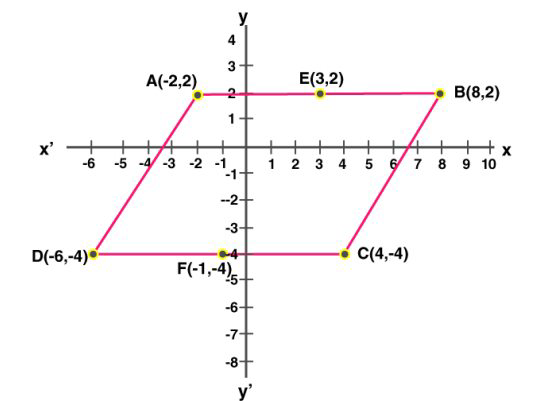# Selina Solutions Class 9 Mathematics Solutions for Exercise 26(A) in Chapter 26 - Chapter 26- Co-ordinate Geometry

A (-2, 2), B (8, 2) and C (4, -4) are the vertices of a parallelogram ABCD. By plotting the given points on a graph paper; find the co-ordinates of the fourth vertex D.

Also, form the same graph, state the co-ordinates of the mid-points of the sides AB and CD

Given, A(2,-2), B(8,2) and C(4,-4) are the vertices of the parallelogram ABCDJoining A, B, C, and D we get the parallelogram ABCD.

According to the graph, we get, D(-6,4)

From the graph,

The coordinates of the mid-point of AB is E(3,2)

The coordinates of the mid-point of CD is F(-1,-4)

Related Questions
Exercises

Lido

Courses

Teachers

Book a Demo with us

Syllabus

Maths
CBSE
Maths
ICSE
Science
CBSE

Science
ICSE
English
CBSE
English
ICSE
Coding

Terms & Policies

Selina Question Bank

Maths
Physics
Biology

Allied Question Bank

Chemistry
Connect with us on social media!